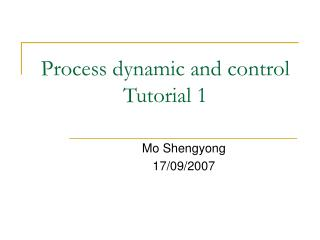DownloadDownload PresentationProcess dynamic and control Tutorial 1

# Process dynamic and control Tutorial 1

Download Presentation## Process dynamic and control Tutorial 1

- - - - - - - - - - - - - - - - - - - - - - - - - - - E N D - - - - - - - - - - - - - - - - - - - - - - - - - - -
##### Presentation Transcript

1. Process dynamic and controlTutorial 1 Mo Shengyong 17/09/2007

2. Self introduction • MoShengyong(莫胜勇) • Tutorial • symo@ust.hk • Lab 7119; Tel 23587146 • Zhou Feng (周峰) • Homework • kebbilay@ust.hk • Lab 7119; Tel 23587146

3. Control valve qw qf, cf q0, cv Example 1 • Consider the following mixing system. Salt solution is fed with qf and cf, and water flow qw. Assume the solution inside the mixer is perfectly mixed. • Control purpose: • To maintain the outlet concentration cv

4. CT CC qw qf, cf q0, cv Question 1 • Propose a feedforward control scheme of cv, by manipulating qf with the control valve • Features: • Disturbance variable is measured • Control variable is not measured

5. CC qw qf, cf CT q0, cv Question 2 • Propose a feedback control scheme • Feedback control features: • Controlled variable is measured • Disturbance variable is not measured

6. Question 3: Compare these two schemes • Feedforward control • Disturbance variable must be measured • No corrective action is taken for unmeasured disturbances • Process model is required • Act before the output is affected • Feedback control • Deal with any kind of disturbance • Act after the output deviates from the set point • Any other better control scheme?

7. + + CC CC CT qw qf, cf CT q0, cv • FF+FB • Feedback control for unmeasured disturbances • Feedforward control for measured disturbances

8. Example 2 • There are two flow control loops. Indicate whether each system is either FB or FF control system. Why? Assume the distance between the flow transmitter and control valve is quite small in each system. FT FC FC FT

9. Answer • Both cases are feedback control • Reason: in both cases, controlled variable (flow rate) is measured, and the controller responds to this measurement.

10. Control valve qw qf, cf q0, cv Example 3 • Establish a dynamic model for the system shown in example 1 • Procedures: see Table 2.1 on page 20

11. Development of dynamic model • Major steps • Modeling objectives • Schematic diagram • Assumptions • Perfect mixing and constant density • Conservation equations • Mass balance • Component balance • Energy balance • Equilibrium equations and simplification • Degrees of freedom analysis • Inputs classification: disturbance or manipulated variable

12. Assumptions: • Perfect mixed • Liquid volume V is constant • Mass balance: